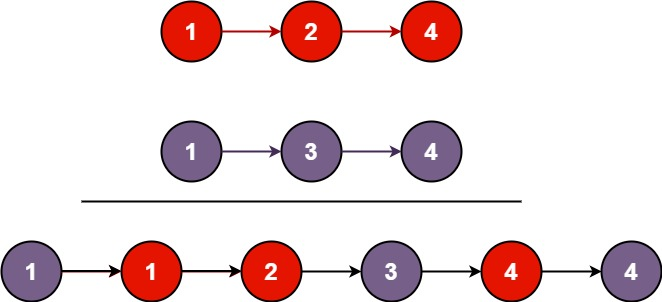# 21.merge-two-sorted-lists

## Statement``````输入：l1 = [1,2,4], l2 = [1,3,4]

``````

``````输入：l1 = [], l2 = []

``````

``````输入：l1 = [], l2 = 

``````

• 两个链表的节点数目范围是 `[0, 50]`
• `-100 <= Node.val <= 100`
• `l1``l2` 均按 非递减顺序 排列

You are given the heads of two sorted linked lists `list1` and `list2`.

Merge the two lists in a one sorted list. The list should be made by splicing together the nodes of the first two lists.

Example 1:``````Input: list1 = [1,2,4], list2 = [1,3,4]
Output: [1,1,2,3,4,4]
``````

Example 2:

``````Input: list1 = [], list2 = []
Output: []
``````

Example 3:

``````Input: list1 = [], list2 = 
Output: 
``````

Constraints:

• The number of nodes in both lists is in the range `[0, 50]`.
• `-100 <= Node.val <= 100`
• Both `list1` and `list2` are sorted in non-decreasing order.

## Solution

``````# Definition for singly-linked list.
# class ListNode:
#     def __init__(self, val=0, next=None):
#         self.val = val
#         self.next = next
from typing import Optional

class Solution:
def mergeTwoLists(self, list1: Optional[ListNode], list2: Optional[ListNode]) -> Optional[ListNode]:
rt = ListNode()
res = rt
while True:
if not list1 and not list2:
break
if not list1:
rt.next = list2
list2 = list2.next
elif not list2:
rt.next = list1
list1 = list1.next
else:
if list1.val < list2.val:
rt.next = list1
list1 = list1.next
else:
rt.next = list2
list2 = list2.next

rt = rt.next
return res.next
``````